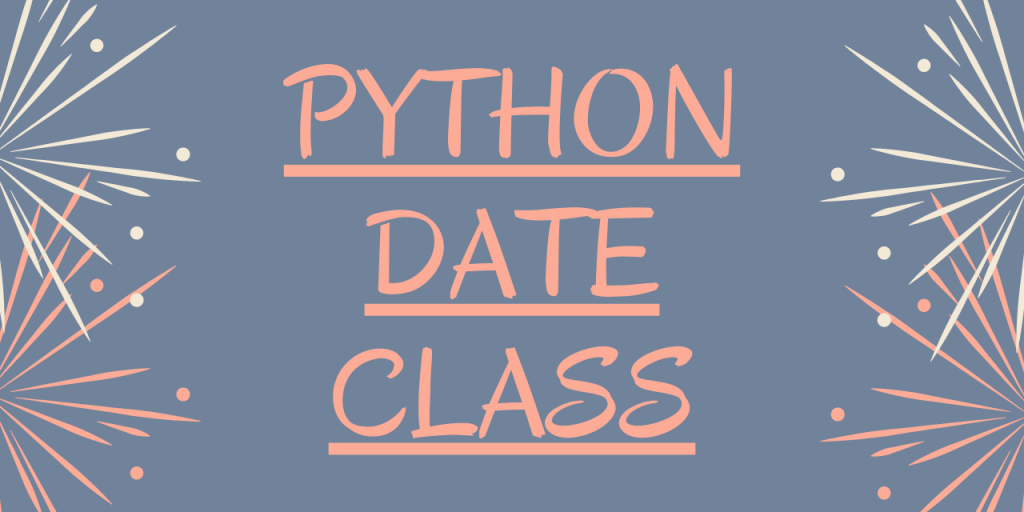# Python date Class – 7 functions to know!Hey! In this article, we will be focusing on Python date class in detail. So, let us get started.

## What is Python datetime module?

Python datetime module offers us with various classes and functions to deal with and manipulate the data values in terms of date and time values.

We need to import the datetime module in order to access the different classes and methods contained in it.

Here, you can use the below snippet of code to import the date class and create a date object through user-defined parameters–

```from datetime import date

dte = date(2020,12,27)

print("Date: ", dte)

```

Output

```Date:  2020-12-27
```

Now, let us understand the date class of Python datetime module in the upcoming section.

## Implementing methods of date class

Python date class contains various methods to work with date format of the data values.

By using the functions of the date class, we can easily manipulate and frame the data values into the standard date format YYYY-MM-DD.

Let us now have a look at some of the important functions framed by the date class function in detail.

### 1. date.today() function

The `date.today() function` fetches the current date from the system and represents the same.

Syntax:

```date.today()
```

Example:

```from datetime import date

dte = date.today()

print("Date: ", dte)
```

Output:

```Date:  2020-07-25
```

### 2. date.year() function

We can access and fetch the year from the date expression using year function. The `year function` extracts and represents the year value from the provided date expression.

Syntax:

```date.today().year
```

Example:

```from datetime import datetime,date

year = date.today().year
print(year)

```

From date.today() function, we get the current date i.e 2020-07-26. Further to which, we have extracted the year value using year() function.

Output:

```2020
```

### 3. date.month() function

In order to extract and represent the month value, `month function `can be used.

Syntax:

```date.today().month
```

Example:

```from datetime import datetime,date

mnth = date.today().month
print(mnth)

```

Output:

```7
```

### 4. date.day() function

The day value from a date expression can be easily extracted using` day function` as shown below–

Syntax:

```date.day
```

Example:

```from datetime import datetime,date

day = date.today().day
print(day)

```

Output: 25

### 5. date.replace() function

At times, a situation may arise, when we would want to alter the date portions of the date expression. This task can be achieved using replace() function.

The `date.replace() function` can be used to replace the below date portions–

• year
• month
• day

Syntax:

```date.replace(year,day,month)
```

Example:

```from datetime import datetime,date

dt = date.today()
print("Current date: ",dt)

res = dt.replace(year=2021,day=20)
print("Modified date: ",res)

```

In the above example, we have replaced the year and day values from the current date (2020-07-26).

Output:

```Current date:  2020-07-26
Modified date:  2021-07-20
```

### 6. date.weekday() function

Using the `weekday function`, we can fetch the weekday number of the day value from the date expression.

The weekdays are provided the index as follows:

• Monday-0
• Tuesday-1
• Wednesday-2
• Thursday-3
• Friday-4
• Saturday-5
• Sunday-6

Example:

```from datetime import datetime,date

date = date.today().weekday()
print("Weekday: ",date)

```

In the above example, we have calculated the weekday number for the current date: 2020-07-26.

Output:

```6
```

### 7. date.strftime() function

The `date.strftime() function` enables us to extract the date portions of the date expressions and represent the values as a String.

To understand the variants of strftime() function, do visit Python strftime() function.

Syntax:

```date.strftime("%Y-%m-%d")
```

Example:

```from datetime import datetime,date

date = date.today()

year = date.strftime("%Y")
print("Current Year:", year)

str_date = date.strftime("%Y-%m-%d")
print("Date value:",str_date)
```

Output:

```Current Year: 2020
Date value: 2020-07-26

```

## Conclusion

By this, we have come to the end of this topic. Feel free to comment below in case you come across any doubt.

Till then, Happy Learning!!